## Static Electricity Review

### Part A: Multiple Choice

1. Which of the following are true of static charges? Choose all that apply.

1. Like charges repel.
2. Like charges attract.
3. Opposite charges repel.
4. Opposite charges attract.
5. A positively charged object has lost electrons.
6. A positively charged object has gained protons.
7. A negatively charged object has lost protons.
8. A negatively charged object has gained electrons.

2. Identify the following objects as being either ...

 a. positive b. negative c. neutral

If there is no conclusive evidence, then select all that could be true.

 Description of Object a, b, or c? i. An object possesses more protons than electrons. ii. An object possesses more neutrons than electrons. iii. A formerly neutral object that just lost some electrons. iv. A formerly neutral object that just gained some electrons. v. An object which attracts a negatively-charged balloon. vi. An object which attracts neutral paper bits and attracts a negatively-charged balloon. vii. An object which attracts neutral attracts paper bits and repels a negatively-charged balloon. viii. An object which attracts a negatively-charged balloon and attracts a positively-charged balloon. ix. An object which attracts a charged balloon (balloon A) which is attracted to a negatively-charged balloon (balloon B). x. An object which attracts a balloon (balloon C) which is repelled by a negatively-charged balloon (balloon D). xi. An object which repels a balloon (balloon E) which is repelled by a positively-charged balloon (balloon F). xii. An object around which the electric field vector is directed inwards. xiii. An object around which the electric field vector is directed outwards.

3. Consider the following statements given below and determine if the charge of Object A is:

 a. positive b. negative c. neutral
 Description of Charging Method a, b, or c? i. Object A is charged by friction using animal fur. Animal fur has a greater electron affinity than object A. ii. Object A is charged by contact using a negatively charged object. iii. Object A is charged by induction using a positively charged object. iv. Object A is used to charge an aluminum plate by the method of induction. The aluminum plate acquires a positive charge. v. Object A is used to charge an aluminum plate by the method of induction. The aluminum plate acquires a negative charge. vi. A rubber rod has a greater electron affinity than animal fur. The rubber rod is charged by friction with animal fur. The rubber rod is then used to charge Object A by the method of contact. vii. A rubber rod has a greater electron affinity than animal fur. The rubber rod is charged by friction with animal fur. The rubber rod is then used to charge Object A by the method of induction. viii. A rubber rod has a greater electron affinity than animal fur. The rubber rod is charged by friction with animal fur. The rubber rod is then used to charge an aluminum pop can by the process of induction. The pop can is then contacted to object A.

4. A neutral plastic strip is rubbed with cotton and acquires a positive charge. Which of the following statements are true of the positively-charged strip?

1. It lost some electrons to the cotton during the charging process.
2. It lost all of its electrons to the cotton during the charging process.
3. It has the opposite charge as the cotton.
4. It would now be repelled by the piece of cotton which was used to charge it.
5. It gained protons during the rubbing process.
6. As a material, plastic has a greater affinity for electrons than cotton.
7. It could exert either a repulsive or attractive influence upon neutral paper bits.
8. It has an excess of protons compared to the number of electrons.
9. It could be used to charge an electroscope negatively by the process of induction.
10. It lost negative electrons and gained positive electrons during the charging process.
11. It lost neutrons during the charging process (or at the very least, its neutrons became ineffective).

5. A positively-charged glass rod is touched to the plate of a neutral electroscope. Upon contact, the electroscope becomes charged and the needle deflects. Which of the following statements are true of the charged electroscope?

1. The electroscope is now charged positively.
2. The electroscope and the glass rod now have the same type of charge.
3. The electroscope was charged by the method of induction.
4. The electroscope gained protons during the charging process.
5. The electroscope gained electrons during the charging process.
6. The electroscope lost all of its electrons during the charging process.
7. During the charging process, some electrons left the electroscope and entered the glass rod.
8. The number of electrons present in the electroscope is less than the number of protons.
9. The electroscope needle will deflect even more if the glass rod is brought near it again.
10. The electroscope needle would slowly approach the neutral position if a negatively-charged balloon is brought near.

6. A negatively-charged balloon is brought near to (without touching) a neutral electroscope. With the negatively-charged balloon held near, the electroscope is momentarily touched by a hand (ground). The balloon is then removed and the needle deflects, thus showing a charge. Which of the following statements are true of the charged electroscope?

1. The electroscope was charged by the method of induction.
2. The charge on the electroscope is the same type of the charge on the balloon.
3. The electroscope has more protons than electrons.
4. The electroscope gained protons during the charging process.
5. The electroscope gained electrons during the charging process.
6. The electroscope lost all of its electrons during the charging process.
7. The electroscope lost some electrons during the charging process.
8. During the charging process, protons moved from the electroscope to the balloon.
9. During the charging process, electrons moved from the electroscope to the balloon.
10. During the charging process, electrons moved from the electroscope to the hand (ground).
11. During the charging process, electrons moved from the hand (ground) to the electroscope.

7. The outer shell electrons in metals are not tightly bound to the nuclei of their atoms. They are free to roam throughout the material, moving from atom to atom. These materials are good ____.

1. conductors
2. insulators
3. for nothing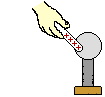For questions #8 and #9, consider the following situation. Connor Duct (Con to his friends) takes a positively charged rubber rod and touches a metal sphere on an insulated stand as shown at the right. Draw the direction of electron flow.

8. The charge on the metal sphere will end up ___.

1. neutral
2. negative
3. positive

9. The sphere acquires this charge because ____.

1. electrons move from the rubber rod to the sphere
2. electrons move from the sphere to the rubber rod
3. protons move from the rubber rod to the sphere
4. protons move from the sphere to the rubber rod
5. the rubber rod creates a charge on the sphere

10. If a positively charged plate is brought near the top of a positively-charged electroscope, then the deflected needle will ____.

1. not move at all
2. be deflected more
3. be deflected less

11. If an electroscope, charged up with excess negative charge, is touched and grounded, then it will end up with ___ charge.

1. no
2. a negative
3. a positive

12. A negatively charged balloon is brought near a metal can that rests on a wood table. The side of the can opposite the balloon is momentarily touched. The can is then _______.

1. positively charged
2. negatively charged
3. uncharged

13. Charge carriers in a metal are electrons rather than protons. This is due to the fact that electrons are __________.

1. loosely bound
2. lighter
3. far from a nucleus
4. all of the above
5. none of the above

14. Two like charges ________.

1. attract each other
2. repel each other
3. neutralize each other
4. have no effect on each other
5. must be neutrons

15. If you comb your hair and the comb becomes positively charged, then your hair becomes _______.

1. positively charged
2. negatively charged
3. uncharged

16. Which of the following charging methods can result in charging an object with a negative charge? Choose all that apply.

 a. charging by friction b. charging by contact c. charging by induction

17. Which of the following charging methods work without ever touching the object to be charged to the object used to charge it? Choose all that apply.

 a. charging by friction b. charging by contact c. charging by induction

18. Which of the following charging methods result in charging an object opposite to the charge on the object used to charge it? Choose all that apply.

 a. charging by friction b. charging by contact c. charging by induction

19. Which of the following statements are true statements about electric force? Choose all that apply.

1. Electric force is a contact force.
2. Electric forces can only act between charged objects - either like-charged or oppositely-charged.
3. Electric forces between two charged objects increases with increasing separation distance.
4. Electric forces between two charged objects increases with increasing quantity of charge on the objects.
5. If object A attracts object B with an electric force, then the attractive force must be mutual - i.e., object B also attracts object A with the same force.
6. A doubling of the quantity of charge on one of the objects results in a doubling of the electric force.
7. A tripling of the quantity of charge on both of the objects results in an increase in the electric force by a factor of 6.
8. A doubling of the separation distance between two point charges results in a quadrupling of the electric force.
9. A tripling of the separation distance between two point charges results in an electric force which is one-sixth of the original value.

20. Which of the following statements are true statements about electric field? Choose all that apply.

1. The electric field strength created by object A is dependent upon the separation distance from object A.
2. The electric field strength created by object A is dependent upon the charge on object A.
3. The electric field strength created by object A is dependent upon the charge of the test object used to measure the strength of the field.
4. The electric field strength about charged Object A is the force per charge experienced by a test charge placed at some location about Object A.
5. As the distance from Object A is doubled, the electric field strength created by object A increases by a factor of 4.
6. As the charge of Object A is doubled, the electric field strength created by object A increases by a factor of 2.
7. As the charge of Object A is doubled and the distance from object A is doubled, the electric field strength created by object A decreases by a factor of 2.
8. Object B is used to test the electric field strength about Object A; as the charge of Object B is doubled, the force which it experiences is doubled but the electric field strength remains the same.
9. Object B is used to test the electric field strength about Object A; as the separation distance between Object A and Object B is doubled, the force which it experiences decreases by a factor of 4 but the electric field strength remains the same.
10. The electric field strength inside of a closed conducting object (e.g., inside the sphere of the Van de Graaff generator) is zero.
11. For irregularly shaped objects, the electric field strength is greatest around points of highest curvature.
12. The electric field is a vector which points in the direction which a positive test charge would be accelerated.
13. Electric fields are directed inwards in regions around negatively-charged objects and outwards in regions around positively-charged objects.
14. Units on electric field are Newtons/Coulomb (N/C).

21. Which of the following statements are true statements about lightning rods? Choose all that apply.

1. Lightning rods are placed on homes to reduce the risk of lightning damage to a home.
2. Any metallic object which is placed on the roof of a home and grounded by an appropriate conducting pathway can serve as a lightning rod.
3. Most lightning rods are pointed as a decorative feature.
4. To be totally effective, a lightning rod must stretch high into the sky and draw charge from the lowest clouds by the method of contact.
5. Lightning rods are capable of reducing the excess charge buildup in clouds, a characteristic of dangerous thunderstorms.
6. Charge is incapable of passing from clouds to a lightning rods since the air between the clouds and the lightning rod has an insulating effect.
7. The electric field strength about the points of lightning rods are very high.

22. Charged balloons are used to induce a charge upon neutral metal pop cans. Identify the type of excess charge which would be present on cans G, H, I, J, K, L, M, N and P as shown in the diagrams below.

 a. positive b. negative c. neutral

 Diagram Type of Charge on... I.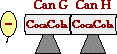G: ____ H: ____ II.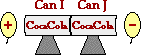I: ____ J: ____ II.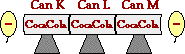K: ____ L: ____ M: ____ IV.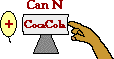N: ____ V.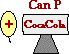P: ____

23. In diagram IV of Question #22, the pop can acquires the charge that it does because ___.

1. electrons move from the balloon to the pop can
2. electrons move from the pop can to the balloon
3. protons move from the balloon to the pop can
4. protons move from the pop can to the balloon
5. electrons move from the pop can to the hand (ground)
6. electrons move from the hand (ground) to the pop can
7. protons move from the pop can to the hand (ground)
8. protons move from the hand (ground) to the pop can

24. A negatively charged balloon will be attracted to a neutral wooden cabinet due to polarization. Which one of the following diagrams best depict why this occurs?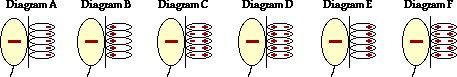For Questions #25 - #27, identify the type of charge on objects A-D based on the electric field lines shown for each configuration of charges.

 a. positive b. negative c. neutral

 25.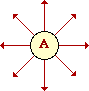26.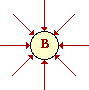27.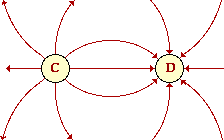### Part B: Diagramming and Analysis

28. A neutral conducting sphere is charged by induction using a positively-charged balloon. What will be the charge of the following sphere in step e if steps a - d are followed? _________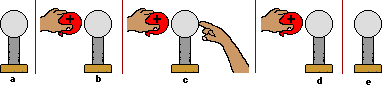Explain how the object acquires this charge. Show the type and location of excess charge on the conducting sphere in each step of the diagram (where appropriate).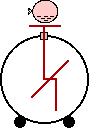29. A negatively-charged balloon is held above (without touching) a neutral electroscope. The presence of the nearby balloon causes the needle of the electroscope to deflect.

a. Draw the location and type of excess charges on the "polarized" neutral electroscope.

b. Explain how the balloon has induced the temporary charge upon the electroscope (i.e., describe the direction of electron movement).

30. Construct electric field lines around the following configuration of charges. Include at least six lines per charge.

 a.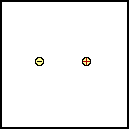b.c.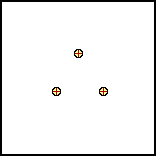d.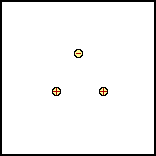31. Use an unbroken arrow to show the direction of electron movement in the following situation. The arrow should extend from the source of the electrons to the final destination of the electrons.

 a. A positively charged balloon is touched to a neutral conducting sphere.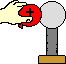b. A glass rod is rubbed with a piece of wool. The wool has the greater electron affinity.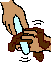c. A positively-charged balloon is held near a neutral conducting sphere. The sphere is then touched on the opposite side.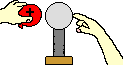d. A negatively-charged rod is held near a neutral conducting sphere. The sphere is touched on the opposite side.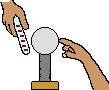32. A negatively-charged object creates an electric field which can be measured at various locations in the region about it. An electric field vector is drawn for point X. Use your understanding of the electric field - distance relationship to draw E vectors for points A - E. (The length of the arrow should be indicative of the relative strength of the E vector.)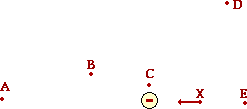### Part C: Short Computations

33. The charge of one electron is -1.6 X 10-19 Coulombs. If a neutral object loses 1.5x106 electrons, then what will be its charge?

34. Express your understanding of Coulomb's law by filling in the following table.

 Q1 (C or µC) Q2 (C or µC) d (m) Felect (N) a. 1.6 x 10-13 C 1.6 x 10-13 C 0.50 m b. 1.6 x 10-7 C 1.6 x 10-7 C 0.017 N c. 4.65 µC 7.28 µC 0.658 m d. 3.21 µC 0.553 m 0.0827 N e. (same as Q1) 1.50 m 4.00 x 10-4 N

35. Charged object A (QA) creates an electric field (E). A positively-charged test charge (qB) is used to measure the electric force (F) at various distances (d) from object A. Express your understanding of electric field strength and electric force by filling in the following table. (Note that the units on charge are fictitious units. Since the value of k is not known in these units, you will have to use your qualitative understanding to answer this question.  That is, you will have to think about relationships - the effect that doubling or quadrupling or halving ... the d or the Q has upon the force and the field strength.)

 QA (bP) qB (bP) d (m) F (mN) E (mN/bP) a. 10.0 0.400 1.00 40.0 b. 10.0 0.800 1.00 c. 10.0 0.400 25.0 d. 20.0 0.400 1.00 200. e. 20.0 0.400 2.00 f. 20.0 0.400 0.500 g. 20.0 1.20 3.00

36. The following questions check your qualitative understanding of the variables effecting electric field and electric force. Use your understanding to fill in the blanks.

1. At a separation distance of 0.500-m, two like-charged balloons experience a repulsive force of 0.320 N. If the distance is doubled, then the repulsive force would be ______ N.
2. At a separation distance of 0.500-m, two like-charged balloons experience a repulsive force of 0.320 N. If the distance is halved, then the repulsive force would be ______ N.
3. At a separation distance of 0.500-m, two like-charged balloons experience a repulsive force of 0.320 N. If the distance is is decreased by a factor of 3 and the charge on one of the balloons is doubled, then the repulsive force would be ______ N.
4. At a separation distance of 0.500-m, two like-charged balloons experience a repulsive force of 0.320 N. If the distance is is increased by a factor of 3 and the charge on both of the balloons is doubled, then the repulsive force would be ______ N.
5. At a location of 0.200 m from object A, the electric field strength is 0.0500 N/C. If the electric field strength were measured at a location of 0.40 m from object A, then the field strength would be _______ N/C.
6. At a location of 0.200 m from object A, the electric field strength is 0.0500 N/C. If the electric field strength were measured at a location of 1.000 m from object A, then the field strength would be _______ N/C
7. At a location of 0.200 m from object A, the electric field strength is 0.0500 N/C. If the electric field strength were measured using a test charge with twice the charge at a location of 0.200 m from object A, then the field strength would be _______ N/C
8. At a location of 0.200 m from object A, the electric field strength is 0.0500 N/C. If the electric field strength were measured using a test charge with twice the charge at a location of 0.100 m from object A, then the field strength would be _______ N/C.

### Part D: Problem-Solving

37. Two objects are located along the y-axis. Object A has a charge of +25 µC and is located at the origin. Object B has a charge of -16 µC and is located a distance of 62 cm above object A. Determine the y-coordinate location where the electric field is zero.

38. Three objects are located along the x-axis. Object A with a charge of +5.6 µC is located at the origin. Object B has a charge of -4.2 µC and is located at the -1.2 m location. Object C has a charge of +7.7 µC and is located at the +2.4 m location. Determine the magnitude and direction of the net electric force acting upon object A.

39. Object A has a x-y coordinate position of (+5.0, 0.0). Object B has a x-y coordinate location of (0.0, +4.0). If object A has a charge of -5.8 µC and object B has a charge of +8.9 µC, then what is the resultant electric field strength at the origin.

40. For the situation described in Question #39, what would be the magnitude of the net electric force upon a +1.3 µC charge if placed at the origin.

41. Alpha particles (i.e., Helium nucleus) have a molar mass of 4.002 g/mol and consist of two protons and two neutrons.

1. Determine the charge of one alpha particle in units of Coulombs and the mass of one alpha particle in units of kg.
2. Suppose that Ernie Rufferthord (not to be confused with the scientist of gold foil fame) wishes to suspend an alpha particle in midair by attracting it to a bundle of electrons held a distance of 1.00-m above the alpha particle. How many electrons would Ernie need in his bundle to accomplish such an amazing feat?

42. A 1.19-gram charged balloon hangs from a 1.99-m string which is attached to the ceiling. A Van de Graaff generator is located directly below the location where the string attaches to the ceiling and is at the same height as the balloon. The string is deflected at an angle of 32.0 degrees from the vertical due to the presence of the electric field. Determine the charge on the Van de Graaff generator if the charge on the balloon is 2.27x10-12 C.

43. Ignoring trace elements, a typical elemental composition (by mass) of the human body is as follows:

 10.5 % H 66.3 % O 19.9 % C 3.3 % N
1. Use these percentages, the molar mass values, Avogadro's number, and the atomic numbers to determine the total number of electrons (and protons) in a 73-kg human body (160 pounds).
2. If these electrons and protons were placed 100 m apart (the distance of approximately one football field), then what would be the force of electrical attraction between them.

#### Navigate to:

Review Session Home - Topic Listing

Static Electricity - Home || Printable Version || Questions with Links

Answers to Questions:  All || #1-16 || #17-32 || #33-43

## You Might Also Like ...

Users of The Review Session are often looking for learning resources that provide them with practice and review opportunities that include built-in feedback and instruction. If that is what you're looking for, then you might also like the following:

The Calculator Pad includes physics word problems organized by topic. Each problem is accompanied by a pop-up answer and an audio file that explains the details of how to approach and solve the problem. It's a perfect resource for those wishing to improve their problem-solving skills.

2. Minds On Physics the App Series

Minds On Physics the App ("MOP the App") is a series of interactive questioning modules for the student that is serious about improving their conceptual understanding of physics. Each module of the series covers a different topic and is further broken down into sub-topics. A "MOP experience" will provide a learner with challenging questions, feedback, and question-specific help in the context of a game-like environment. It is available for phones, tablets, Chromebooks, and Macintosh computers. It's a perfect resource for those wishing to refine their conceptual reasoning abilities. Part 4 of the series includes topics in Static Electricity.

Visit: MOP the App Home || MOP the App - Part 4